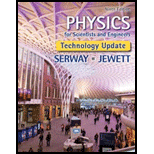# A 50.0-g object connected to a spring with a force constant of 35.0 N/m oscillates with an amplitude of 4.00 cm on a frictionless, horizontal surface. Find (a) the total energy of the system and (b) the speed of the object when its position is 1.00 cm. Find (c) the kinetic energy and (d) the potential energy when its position is 3.00 cm.### Physics for Scientists and Enginee...

9th Edition
Raymond A. Serway + 1 other
Publisher: Cengage Learning
ISBN: 9781305116399

#### Solutions

Chapter
Section### Physics for Scientists and Enginee...

9th Edition
Raymond A. Serway + 1 other
Publisher: Cengage Learning
ISBN: 9781305116399
Chapter 15, Problem 15.27P
Textbook Problem
22 views

## A 50.0-g object connected to a spring with a force constant of 35.0 N/m oscillates with an amplitude of 4.00 cm on a frictionless, horizontal surface. Find (a) the total energy of the system and (b) the speed of the object when its position is 1.00 cm. Find (c) the kinetic energy and (d) the potential energy when its position is 3.00 cm.

(a)

To determine

The total energy of the system.

### Explanation of Solution

Given info: The spring constant is 35.0N/m , the amplitude of motion is 4.00cm and the mass of the block is 50.0g .

Write the expression for the total energy.

E=kA22

Here,

E is the distance covered by the rocket during the negative acceleration.

A is the amplitude of the motion.

Substitute 4.00cm for A and 35.0N/m for k in above equation.

E=(35

(b)

To determine

The speed of the object when its position is 1.00cm .

(c)

To determine

The kinetic energy when the position of the object is 3.00cm .

(d)

To determine

The potential energy when the position of the object is 3.00cm .

### Still sussing out bartleby?

Check out a sample textbook solution.

See a sample solution

#### The Solution to Your Study Problems

Bartleby provides explanations to thousands of textbook problems written by our experts, many with advanced degrees!

Get Started

Find more solutions based on key concepts
How does a tendon differ from a ligament?

Human Biology (MindTap Course List)

Name the following compounds. a CaO b Mn2O3 c NH4HCO3 d Cu(NO3)2

General Chemistry - Standalone book (MindTap Course List)

What is the difference between artificial selection and natural selection?

Biology: The Dynamic Science (MindTap Course List)

2. Describe an atom.

Chemistry In Focus

Which of the following is a physical consequence of fasting? a. loss of lean body tissues b. lasting weight los...

Nutrition: Concepts and Controversies - Standalone book (MindTap Course List)

Calculate the age of the Grand Canyon as a fraction of Earths age.

Foundations of Astronomy (MindTap Course List)

How are sea turtles able to find their way back to the beach where they originally hatched?

Oceanography: An Invitation To Marine Science, Loose-leaf Versin